# Bayes Factors

This vignette can be referred to by citing the following:

• Makowski, D., Ben-Shachar, M. S., & Lüdecke, D. (2019). bayestestR: Describing Effects and their Uncertainty, Existence and Significance within the Bayesian Framework. Journal of Open Source Software, 4(40), 1541. https://doi.org/10.21105/joss.01541

• Makowski, D., Ben-Shachar, M. S., Chen, S. H. A., & Lüdecke, D. (2019). Indices of Effect Existence and Significance in the Bayesian Framework. Retrieved from 10.3389/fpsyg.2019.02767

The adoption of the Bayesian framework for applied statistics, especially in the social and psychological sciences, seems to be developing in two distinct directions. One of the key topics marking their separation is their opinion about the Bayes factor. In short, one school of thought (e.g., the Amsterdam school, led by E. J. Wagenmakers) advocate its use, and emphasize its qualities as a statistical index, while another point to its limits and prefer, instead, the precise description of posterior distributions (using CIs, ROPEs, etc.).

The bayestestR package does not take a side in this debate, and offers tools to carry out analysis irrespective of the school you subscribe to. Instead, it strongly supports the notion of an informed choice:

discover the methods, learn about them, understand them, try them, and decide for yourself.

Having said that, here’s an introduction to Bayes factors :)

# The Bayes Factor

Bayes Factors (BFs) are indices of relative evidence of one “model” over another.

In their role as a hypothesis testing index, they are to Bayesian framework what a $$p$$-value is to the classical/frequentist framework. In significance-based testing, $$p$$-values are used to assess how unlikely are the observed data if the null hypothesis were true, while in the Bayesian model selection framework, Bayes factors assess evidence for different models, each model corresponding to a specific hypothesis.

According to Bayes’ theorem, we can update prior probabilities of some model $$M$$ ($$P(M)$$) to posterior probabilities ($$P(M|D)$$) after observing some datum $$D$$ by accounting for the probability of observing that datum given the model ($$P(D|M)$$, also known as the likelihood):

$P(M|D) = \frac{P(D|M)\times P(M)}{P(D)}$

Using this equation, we can compare the probability-odds of two models:

$\underbrace{\frac{P(M_1|D)}{P(M_2|D)}}_{\text{Posterior Odds}} = \underbrace{\frac{P(D|M_1)}{P(D|M_2)}}_{\text{Likelihood Ratio}} \times \underbrace{\frac{P(M_1)}{P(M_2)}}_{\text{Prior Odds}}$

Where the likelihood ratio (the middle term) is the Bayes factor - it is the factor by which some prior odds have been updated after observing the data to posterior odds.

Thus, Bayes factors can be calculated in two ways:

• As a ratio quantifying the relative probability of the observed data under each of the two models. (In some contexts, these probabilities are also called marginal likelihoods.)

$BF_{12}=\frac{P(D|M_1)}{P(D|M_2)}$

• As the degree of shift in prior beliefs about the relative credibility of two models (since they can be computed by dividing posterior odds by prior odds).

$BF_{12}=\frac{Posterior~Odds_{12}}{Prior~Odds_{12}}$

Here we provide functions for computing Bayes factors in two different contexts:

• testing single parameters (coefficients) within a model
• comparing statistical models themselves

# Testing Models’ Parameters with Bayes Factors

A Bayes factor for a single parameter can be used to answer the question:

“Given the observed data, has the null hypothesis of an absence of an effect become more or less credible?”Bayesian analysis of the Students’ (1908) Sleep data set.

Let’s use the Students’ (1908) Sleep data set (data("sleep")). The data comes from a study in which participants were administered a drug and the researchers assessed the extra hours of sleep that participants slept afterwards. We will try answering the following research question using Bayes factors:

Given the observed data, has the hypothesis that the drug (the effect of group) has no effect on the numbers of hours of extra sleep (variable extra) become more of less credible?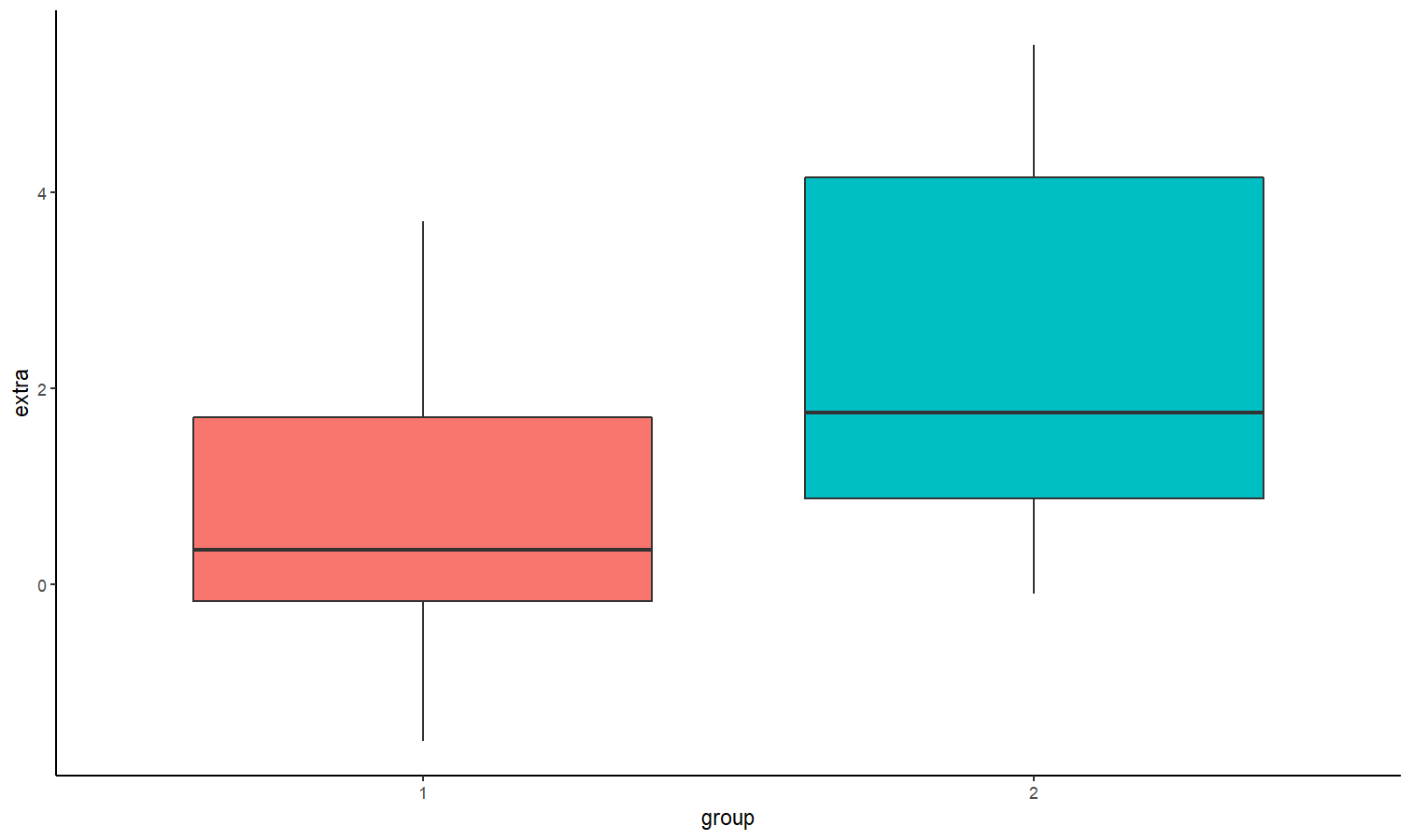The boxplot suggests that the second group has a higher number of hours of extra sleep. By how much?

Let’s fit a simple Bayesian linear model, with a prior of $$b_{group} \sim N(0, 3)$$ (i.e. the prior follows a Gaussian/normal distribution with $$mean = 0$$ and $$SD = 3$$), using rstanarm package:

set.seed(123)
library(rstanarm)

model <- stan_glm(
formula = extra ~ group,
data = sleep,
prior = normal(0, 3, autoscale = FALSE)
)

### Testing against a null-region

One way of operationlizing the null-hypothesis is by setting a null region, such that an effect that falls within this interval would be practically equivalent to the null (Kruschke, 2010). In our case, that means defining a range of effects we would consider equal to the drug having no effect at all. We can then compute the prior probability of the drug’s effect falling within this null-region, and the prior probability of the drug’s effect falling outside the null-region to get our prior odds. Say any effect smaller than an hour of extra sleep is practically equivalent to being no effect at all, we would define our prior odds as:

$\frac {P(b_{drug} \in [-1, 1])} {P(b_{drug} \notin [-1, 1])}$

Given our prior has a normal distribution centered at 0 hours with a scale (an SD) of 3 hours, our priors would look like this: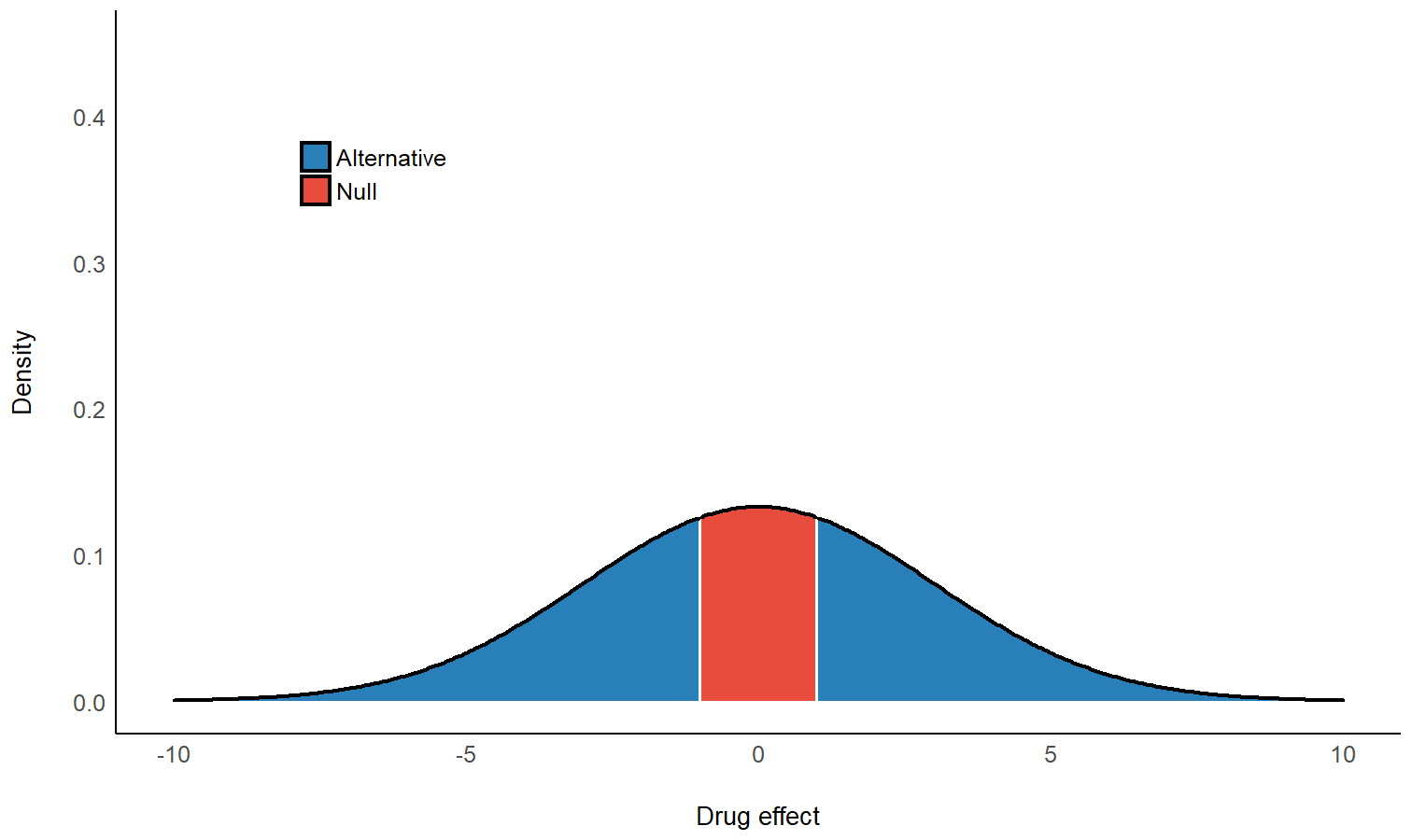and the prior odds would be 2.2.

By looking at the posterior distribution, can now compute the posterior probability of the drug’s effect falling within the null-region, and the posterior probability of the drug’s effect falling outside the null-region to get our posterior odds:

$\frac {P(b_{drug} \in [-1,1] | Data)} {P(b_{drug} \notin [-1,1] | Data)}$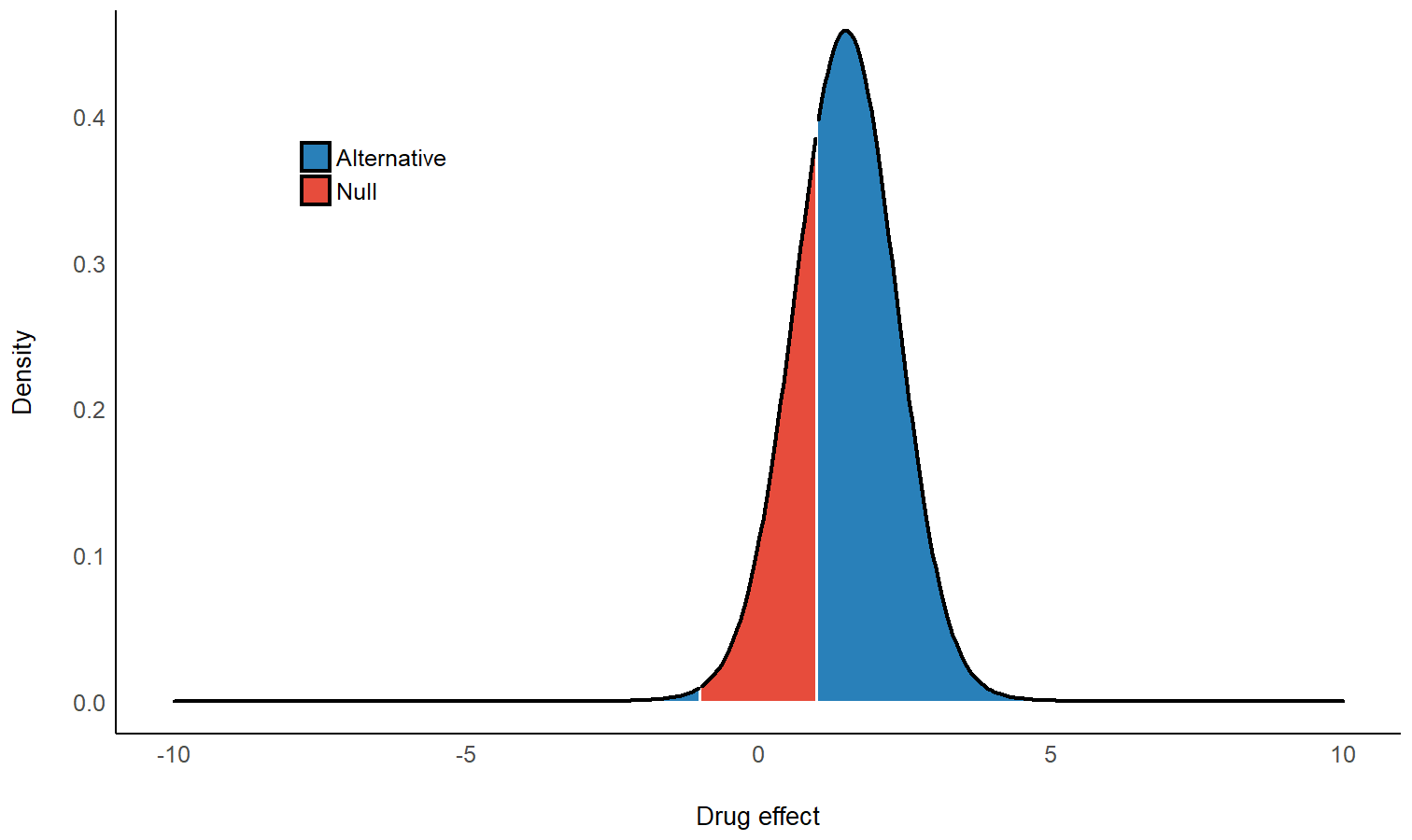We can see that the center of the posterior distribution has shifted away from 0 (to ~1.5). Likewise, the posterior odds are 2, which seems to favor the effect being non-null. But, does this mean the data support the alternative over the null? Hard to say, since even before the data were observed, the priors already favored the alternative - so we need to take our priors into account here!

Let’s compute the Bayes factor as the change from the prior odds to the posterior odds: $$BF_{10} = Odds_{posterior} / Odds_{prior} = 0.9$$! This BF indicates that the data provide 1/0.9 = 1.1 times more evidence for the effect of the drug being practically nothing than it does for the drug having some clinically significant effect. Thus, although the center of distribution has shifted away from 0, and the posterior distribution seems to favor a non-null effect of the drug, it seems that given the observed data, the probability mass has overall shifted closer to the null interval, making the values in the null interval more probable! (see Non-overlapping Hypotheses in Morey & Rouder, 2011)

All of this can be achieved with the function bayesfactor_parameters(), which computes a Bayes factor for each of the model’s parameters:

My_first_BF <- bayesfactor_parameters(model, null = c(-1, 1))
My_first_BF
> Bayes Factor (Null-Interval)
>
> Parameter   |    BF
> -------------------
> (Intercept) | 0.103
> group2      | 0.883
>
> * Evidence Against The Null: [-1.000, 1.000]

We can also plot using the see package:

library(see)
plot(My_first_BF)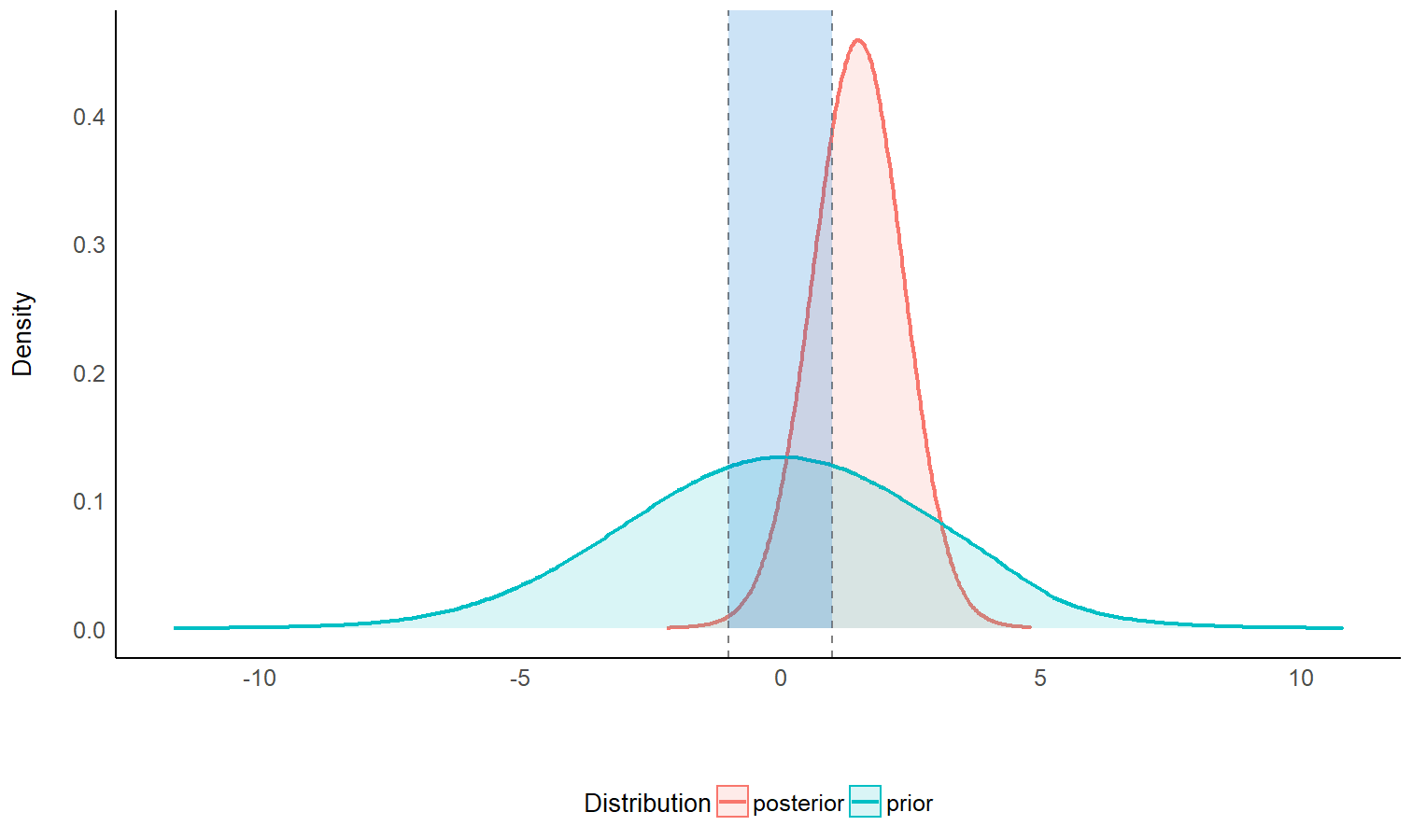Note that interpretation guides for Bayes factors can be found in the effectsize package:

effectsize::interpret_bf(exp(My_first_BF$log_BF), include_value = TRUE) >  "anecdotal evidence (BF = 1/1.13) against" > (Rules: jeffreys1961) ### Testing against the point-null (0) What if we don’t know what region would be practically equivalent to 0? Or if we just want the null to be exactly zero? Not a problem - as the width of null region shrinks to a point, the change from the prior probability to the posterior probability of the null can be estimated by comparing the density of the null value between the two distributions.1 This ratio is called the Savage-Dickey ratio, and has the added benefit of also being an approximation of a Bayes factor comparing the estimated model against the a model in which the parameter of interest has been restricted to a point-null: “[…] the Bayes factor for $$H_0$$ versus $$H_1$$ could be obtained by analytically integrating out the model parameter $$\theta$$. However, the Bayes factor may likewise be obtained by only considering $$H_1$$, and dividing the height of the posterior for $$\theta$$ by the height of the prior for $$\theta$$, at the point of interest.” (Wagenmakers, Lodewyckx, Kuriyal, & Grasman, 2010) My_second_BF <- bayesfactor_parameters(model, null = 0) My_second_BF > Bayes Factor (Savage-Dickey density ratio) > > Parameter | BF > ---------------- > group2 | 1.24 > > * Evidence Against The Null: 0 plot(My_second_BF)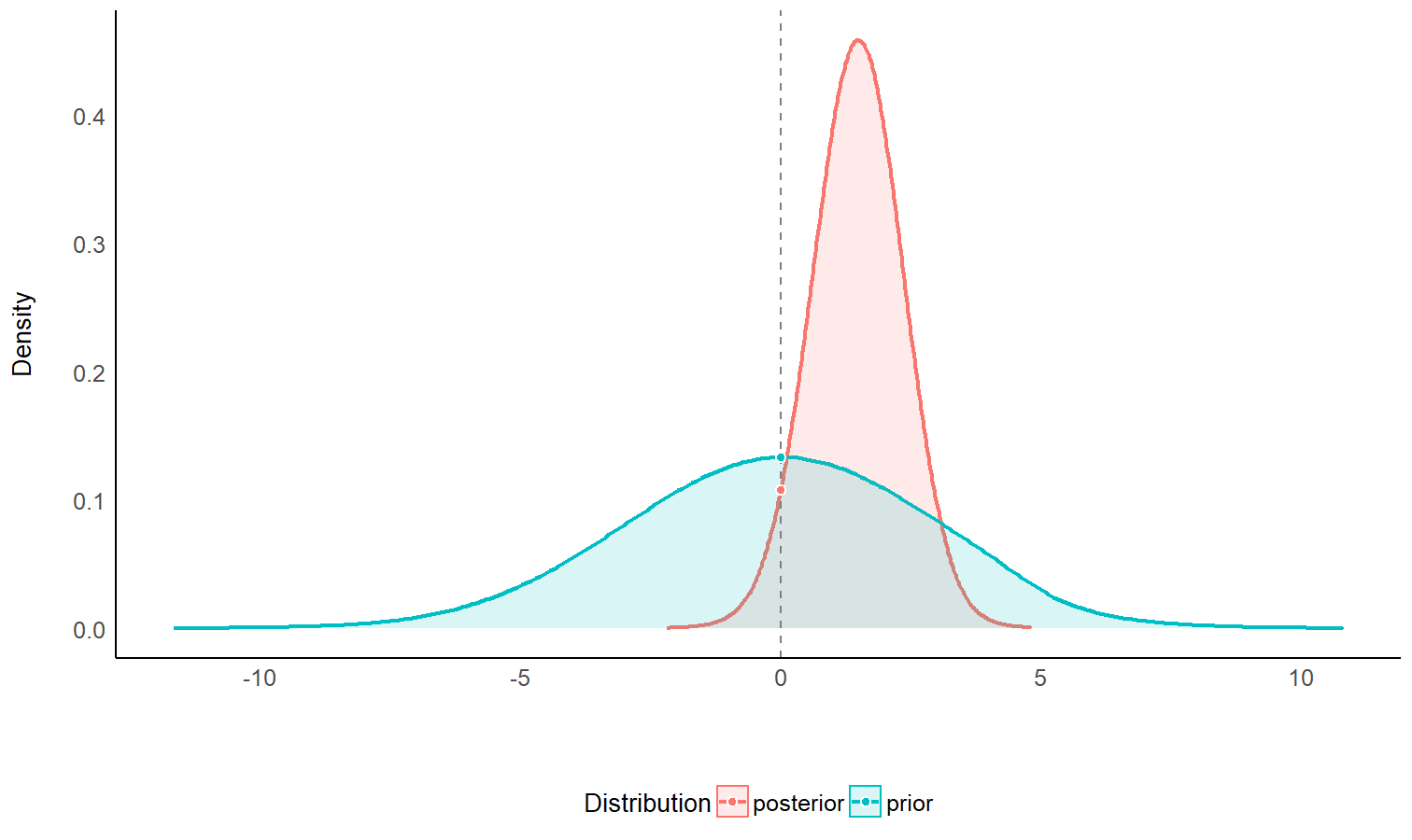### Directional hypotheses We can also compute Bayes factors for directional hypotheses (“one sided”), if we have a prior hypotheses about the direction of the effect. This can be done by setting an order restriction on the prior distribution (which results in an order restriction on the posterior distribution) of the alternative (Morey & Wagenmakers, 2014). For example, if we have a prior hypothesis that the drug has a positive effect on the number of sleep hours, the alternative will be restricted to the region to the right of the null (point or interval): test_group2_right <- bayesfactor_parameters(model, direction = ">") test_group2_right > Bayes Factor (Savage-Dickey density ratio) > > Parameter | BF > ---------------- > group2 | 2.37 > > * Evidence Against The Null: 0 > * Direction: Right-Sided test plot(test_group2_right)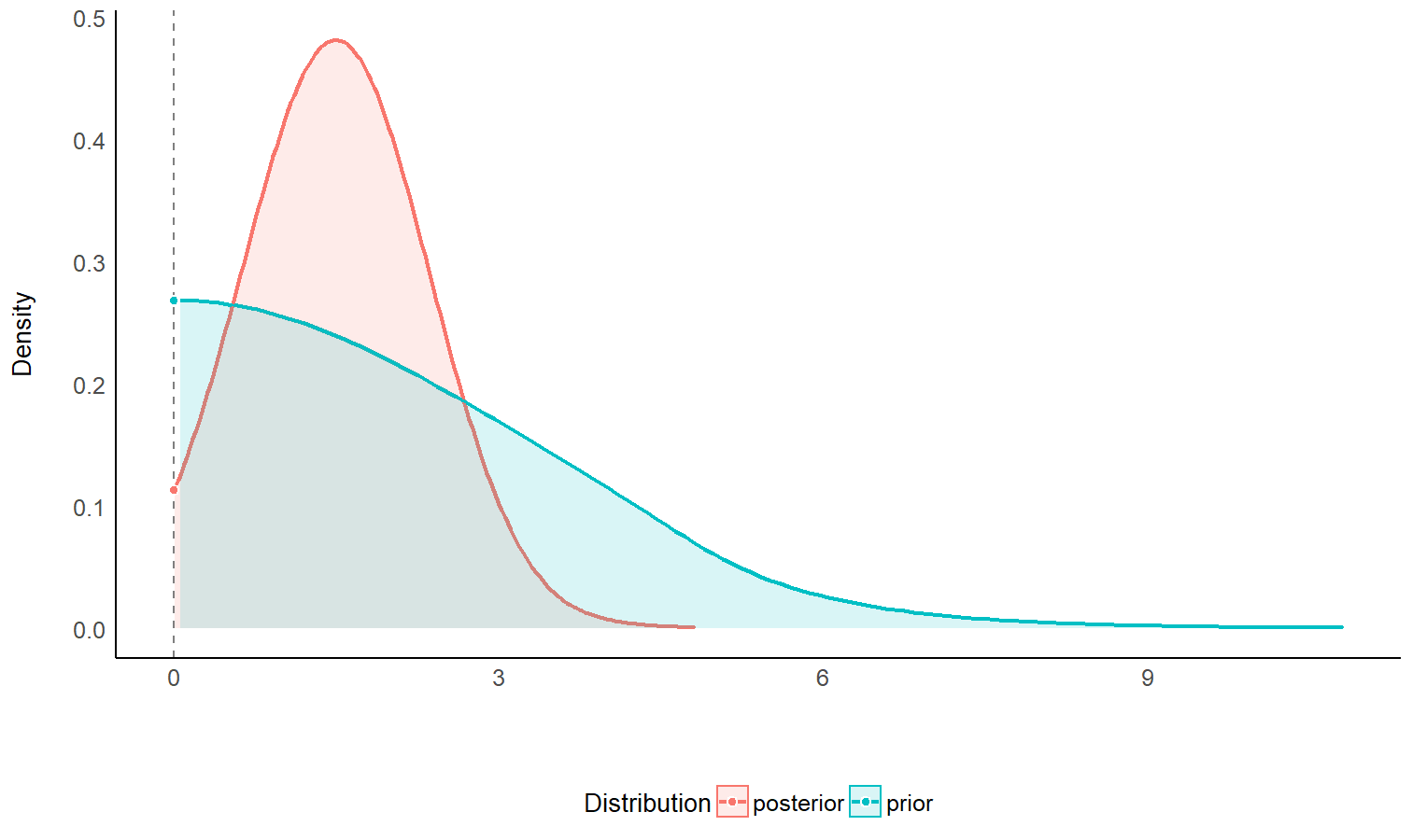As we can see, given that we have an a priori assumption about the direction of the effect (that the effect is positive), the presence of an effect is 2.8 times more likely than the absence of an effect, given the observed data (or that the data are 2.8 time more probable under $$H_1$$ than $$H_0$$). This indicates that, given the observed data, and a priori hypothesis, the posterior mass has shifted away from the null value, giving some evidence against the null (note that a Bayes factor of 2.8 is still considered quite weak evidence). Thanks to the flexibility of Bayesian framework, it is also possible to compute a Bayes factor for dividing hypotheses - that is, for a null and alternative that are complementary, opposing one-sided hypotheses (Morey & Wagenmakers, 2014). For example, above we compared an alternative of $$H_A$$: the drug has a positive effects to the null $$H_0$$: the drug has no effect. But we can also compare instead the same alternative to its complementary hypothesis: $$H_{-A}$$: the drug has a negative effects. test_group2_dividing <- bayesfactor_parameters(model, null = c(-Inf, 0)) test_group2_dividing > Bayes Factor (Null-Interval) > > Parameter | BF > ----------------- > group2 | 20.53 > > * Evidence Against The Null: [-Inf, 0.000] plot(test_group2_dividing)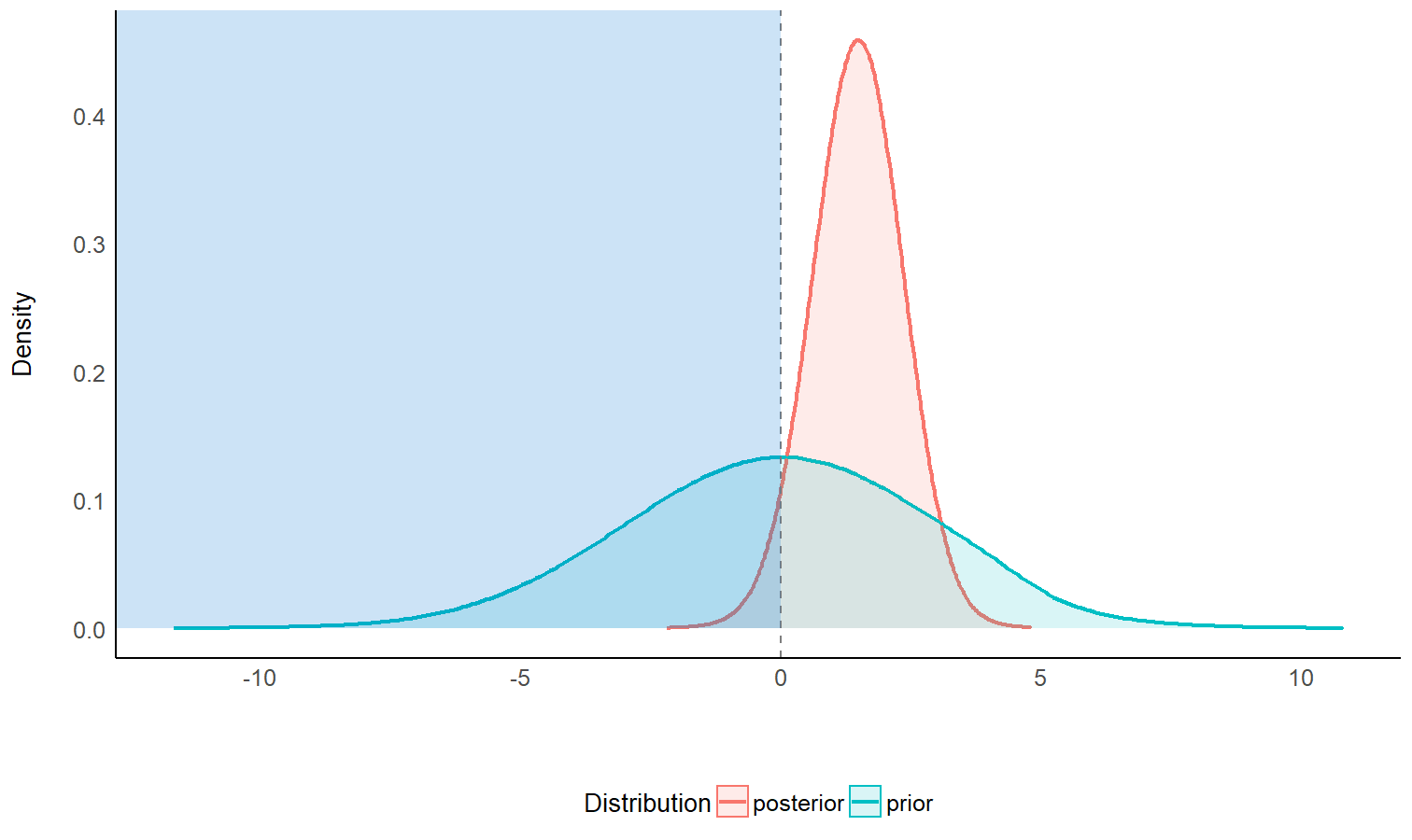We can see that this test produces even stronger (more conclusive) evidence than the one-sided vs. point-null test! And indeed, as a rule of thumb, the more specific the two hypotheses are, and the more distinct they are from one another, the more power our Bayes factor has!2 Thanks to the transitivity of Bayes factors, we can also use bayesfactor_parameters() to compare even more types of hypotheses, with some trickery. For example: $\underbrace{BF_{0<b<1\text{ vs. }b=0}}_{\text{range vs. point}} = \underbrace{BF_{b<0\text{ vs. }b=0}}_{\text{directional vs. point}} / \underbrace{BF_{b<0\text{ vs. }0<b<1}}_{\text{directional vs. range}}$ NOTE: See the Testing Contrasts appendix below. ### Support intervals So far we’ve seen that Bayes factors quantify relative support between competing hypotheses. However, we can also ask: Upon observing the data, the credibility of which of the parameter’s values has increased (or decreased)? For example, we’ve seen that the point null has become somewhat less credible after observing the data, but we might also ask which values have gained credibility given the observed data?. The resulting range of values is called the support interval as it indicates which values are supported by the data (Wagenmakers, Gronau, Dablander, & Etz, 2018). We can do this by once again comparing the prior and posterior distributions and checking where the posterior densities are higher than the prior densities. In bayestestR, this can be achieved with the si() function: my_first_si <- si( posterior = data.frame(group2 = posterior), prior = data.frame(group2 = prior), BF = 1 ) print(my_first_si) > Support Interval > > Parameter | BF = 1 SI > ------------------------ > group2 | [0.15, 3.04] The argument BF = 1 indicates that we want the interval to contain values that have gained support by a factor of at least 1 (that is, any support at all). Visually, we can see that the credibility of all the values within this interval has increased (and likewise the credibility of all the values outside this interval has decreased): plot(my_first_si)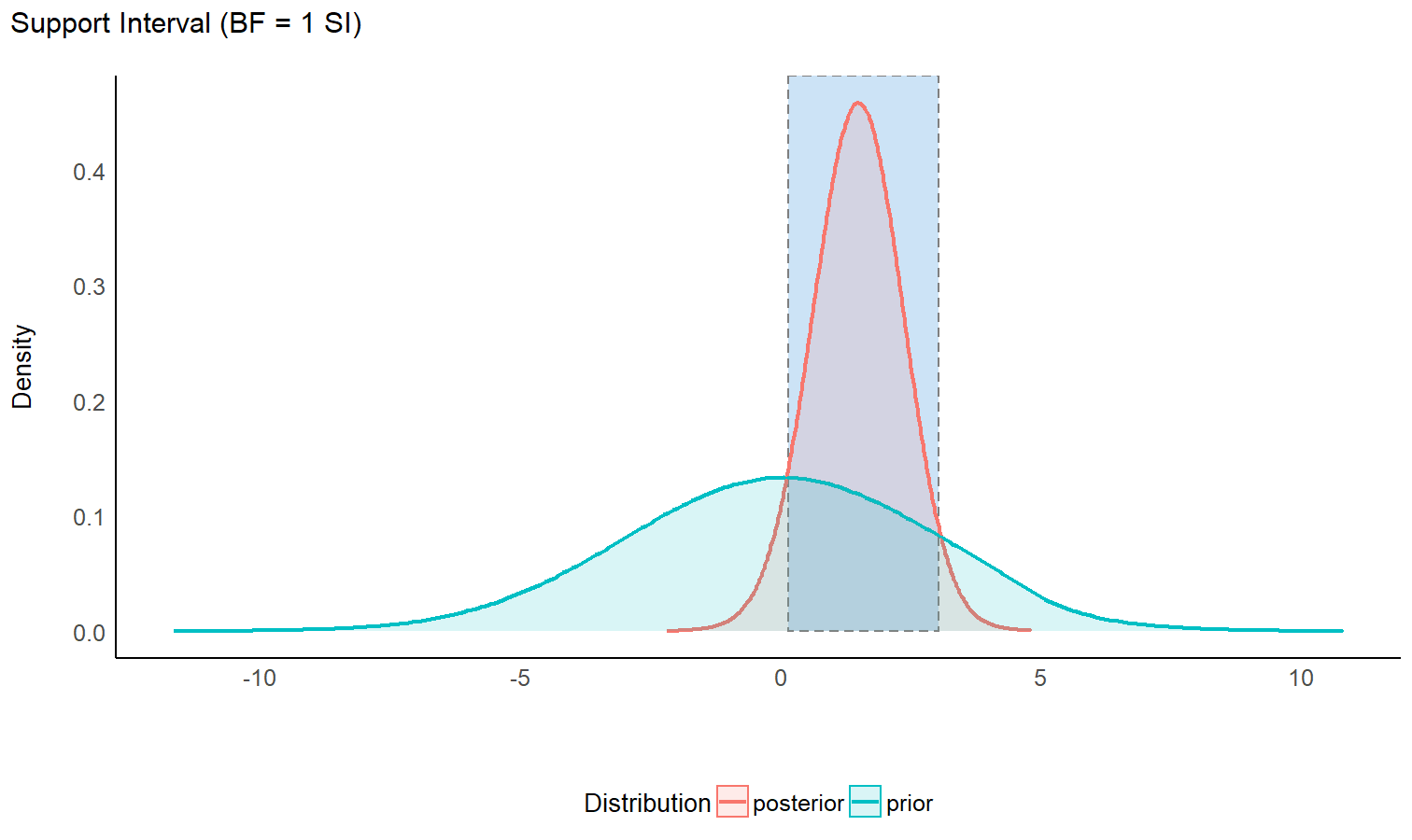We can also see the this support interval (just barely) excludes the point null (0) - whose credibility we’ve already seen has decreased by the observed data. This emphasizes the relationship between the support interval and the Bayes factor: “The interpretation of such intervals would be analogous to how a frequentist confidence interval contains all the parameter values that would not have been rejected if tested at level $$\alpha$$. For instance, a BF = 1/3 support interval encloses all values of theta for which the updating factor is not stronger than 3 against.” (Wagenmakers et al., 2018) Thus, the choice of BF (the level of support the interval should indicate) depends on what we want our interval to represent: • A $$BF = 1$$ contains values whose credibility has merely not decreased by observing the data. • A $$BF > 1$$ contains values who received more impressive support from the data. • A $$BF < 1$$ contains values whose credibility has not been impressively decreased by observing the data. Testing against values outside this interval will produce a Bayes factor larger than $$1/BF$$ in support of the alternative. # Comparing Models using Bayes Factors Bayes factors can also be used to compare statistical models. In this statistical context, they answer the following question: Under which model are the observed data more probable? In other words, which model is more likely to have produced the observed data? This is usually done by comparing the marginal likelihoods of two models. In such a case, the Bayes factor is a measure of the relative evidence for one model over the other. Let’s use Bayes factors for model comparison to find a model that best describes the length of an iris’ sepal using the iris data set. ### For Bayesian models (brms and rstanarm) Note: In order to compute Bayes factors for Bayesian models, non-default arguments must be added upon fitting: • brmsfit models must have been fitted with save_pars = save_pars(all = TRUE) • stanreg models must have been fitted with a defined diagnostic_file. Let’s first fit 5 Bayesian regressions with brms to predict Sepal.Length: library(brms) # intercept only model m0 <- brm(Sepal.Length ~ 1, data = iris, prior = set_prior("student_t(3, 6, 6)", class = "Intercept") + set_prior("student_t(3, 0, 6)", class = "sigma"), save_pars = save_pars(all = TRUE), backend = "rstan") # Petal.Length only m1 <- brm(Sepal.Length ~ Petal.Length, data = iris, prior = set_prior("student_t(3, 6, 6)", class = "Intercept") + set_prior("student_t(3, 0, 6)", class = "sigma") + set_prior("normal(0, 1)", coef = "Petal.Length"), save_pars = save_pars(all = TRUE)) # Species only m2 <- brm(Sepal.Length ~ Species, data = iris, prior = set_prior("student_t(3, 6, 6)", class = "Intercept") + set_prior("student_t(3, 0, 6)", class = "sigma") + set_prior("normal(0, 3)", coef = c("Speciesversicolor", "Speciesvirginica")), save_pars = save_pars(all = TRUE)) # Species + Petal.Length model m3 <- brm(Sepal.Length ~ Species + Petal.Length, data = iris, prior = set_prior("student_t(3, 6, 6)", class = "Intercept") + set_prior("student_t(3, 0, 6)", class = "sigma") + set_prior("normal(0, 1)", coef = "Petal.Length") + set_prior("normal(0, 3)", coef = c("Speciesversicolor", "Speciesvirginica")), save_pars = save_pars(all = TRUE)) # full interactive model m4 <- brm(Sepal.Length ~ Species * Petal.Length, data = iris, prior = set_prior("student_t(3, 6, 6)", class = "Intercept") + set_prior("student_t(3, 0, 6)", class = "sigma") + set_prior("normal(0, 1)", coef = "Petal.Length") + set_prior("normal(0, 3)", coef = c("Speciesversicolor", "Speciesvirginica")) + set_prior("normal(0, 2)", coef = c("Speciesversicolor:Petal.Length", "Speciesvirginica:Petal.Length")), save_pars = save_pars(all = TRUE)) We can now compare these models with the bayesfactor_models() function, using the denominator argument to specify the model against which the rest of the models will be compared (in this case, the intercept-only model): library(bayestestR) comparison <- bayesfactor_models(m1, m2, m3, m4, denominator = m0) comparison > Bayes Factors for Model Comparison > > Model BF >  Petal.Length 1.27e+44 >  Species 8.34e+27 >  Species + Petal.Length 2.29e+53 >  Species * Petal.Length 9.79e+51 > > * Against Denominator:  (Intercept only) > * Bayes Factor Type: marginal likelihoods (bridgesampling) We can see that the Species + Petal.Length model is the best model - with $$BF=2\times 10^{53}$$ compared to the null (intercept only). Due to the transitive property of Bayes factors, we can easily change the reference model to the full Species * Petal.Length model: update(comparison, reference = 4) > Bayes Factors for Model Comparison > > Model BF >  Petal.Length 1.30e-08 >  Species 8.52e-25 >  Species + Petal.Length 23.38 >  (Intercept only) 1.02e-52 > > * Against Denominator:  Species * Petal.Length > * Bayes Factor Type: marginal likelihoods (bridgesampling) As we can see, the Species + Petal.Length model is also favored compared to the Species * Petal.Length model, though to several orders of magnitude less - is is only supported 23.38 times more!) We can also change the reference model to the Species model: update(comparison, reference = 2) > Bayes Factors for Model Comparison > > Model BF >  Petal.Length 1.53e+16 >  Species + Petal.Length 2.74e+25 >  Species * Petal.Length 1.17e+24 >  (Intercept only) 1.20e-28 > > * Against Denominator:  Species > * Bayes Factor Type: marginal likelihoods (bridgesampling) Notice that, in the Bayesian framework the compared models do not need to be nested models, as happened here when we compared the Petal.Length-only model to the Species-only model (something that cannot be done in the frequentist framework, where compared models must be nested in one another). We can also get a matrix of Bayes factors of all the pairwise model comparisons: as.matrix(comparison) > # Bayes Factors for Model Comparison > > Numerator > Denominator > >  |  |  |  |  |  > --------------------------------------------------------------------------------- >  Petal.Length | 1 | 6.54e-17 | 1.80e+09 | 7.68e+07 | 7.85e-45 >  Species | 1.53e+16 | 1 | 2.74e+25 | 1.17e+24 | 1.20e-28 >  Species + Petal.Length | 5.57e-10 | 3.64e-26 | 1 | 0.043 | 4.37e-54 >  Species * Petal.Length | 1.30e-08 | 8.52e-25 | 23.38 | 1 | 1.02e-52 >  (Intercept only) | 1.27e+44 | 8.34e+27 | 2.29e+53 | 9.79e+51 | 1 NOTE: In order to correctly and precisely estimate Bayes Factors, you always need the 4 P’s: Proper Priors,3 and a Plentiful Posterior.4 ### For Frequentist models via the BIC approximation It is also possible to compute Bayes factors for the comparison of frequentist models. This is done by comparing BIC measures, allowing a Bayesian comparison of non-nested frequentist models (Wagenmakers, 2007). Let’s try it out on some linear mixed-effects models: library(lme4) # define models with increasing complexity m0 <- lmer(Sepal.Length ~ (1 | Species), data = iris) m1 <- lmer(Sepal.Length ~ Petal.Length + (1 | Species), data = iris) m2 <- lmer(Sepal.Length ~ Petal.Length + (Petal.Length | Species), data = iris) m3 <- lmer(Sepal.Length ~ Petal.Length + Petal.Width + (Petal.Length | Species), data = iris) m4 <- lmer(Sepal.Length ~ Petal.Length * Petal.Width + (Petal.Length | Species), data = iris) # model comparison bayesfactor_models(m1, m2, m3, m4, denominator = m0) > Bayes Factors for Model Comparison > > Model BF > [m1] Petal.Length + (1 | Species) 8.24e+24 > [m2] Petal.Length + (Petal.Length | Species) 4.77e+23 > [m3] Petal.Length + Petal.Width + (Petal.Length | Species) 1.52e+22 > [m4] Petal.Length * Petal.Width + (Petal.Length | Species) 5.93e+20 > > * Against Denominator: [m0] 1 + (1 | Species) > * Bayes Factor Type: BIC approximation ### Order restricted models As stated above when discussing one-sided hypothesis tests, we can create new models by imposing order restrictions on a given model. For example, consider the following model, in which we predict the length of an iris’ sepal from the length of its petal, as well as from its species, with priors: - $$b_{petal} \sim N(0,2)$$ - $$b_{versicolors}\ \&\ b_{virginica} \sim N(0,1.2)$$ iris_model <- stan_glm(Sepal.Length ~ Species + Petal.Length, data = iris, prior = normal(0, c(2, 1.2, 1.2), autoscale = FALSE), refresh = 0 ) These priors are unrestricted - that is, all values between $$-\infty$$ and $$\infty$$ of all parameters in the model have some non-zero credibility (no matter how small; this is true for both the prior and posterior distribution). Subsequently, a priori the ordering of the parameters relating to the iris species can have any ordering, such that a priori setosa can have larger sepals than virginica, but it is also possible for virginica to have larger sepals than setosa! Does it make sense to let our priors cover all of these possibilities? That depends on our prior knowledge or hypotheses. For example, even a novice botanist will assume that it is unlikely that petal length will be negatively associated with sepal length - an iris with longer petals is likely larger, and thus will also have a longer sepal. And an expert botanist will perhaps assume that setosas have smaller sepals than both versicolors and virginica. These priors can be formulated as restricted priors (Morey, 2015; Morey & Rouder, 2011): 1. The novice botanist: $$b_{petal} > 0$$ 2. The expert botanist: $$b_{versicolors} > 0\ \&\ b_{virginica} > 0$$ By testing these restrictions on prior and posterior samples, we can see how the probabilities of the restricted distributions change after observing the data. This can be achieved with bayesfactor_restricted(), that compute a Bayes factor for these restricted model vs the unrestricted model. Let’s first specify these restrictions as logical conditions: botanist_hypotheses <- c( "Petal.Length > 0", "(Speciesversicolor > 0) & (Speciesvirginica > 0)" ) Let’s test these hypotheses: model_prior <- unupdate(iris_model) botanist_BFs <- bayesfactor_restricted( posterior = iris_model, prior = model_prior, hypothesis = botanist_hypotheses ) print(botanist_BFs) > Bayes Factor (Order-Restriction) > > Hypothesis P(Prior) P(Posterior) BF > Petal.Length > 0 0.50 1 2.02 > (Speciesversicolor > 0) & (Speciesvirginica > 0) 0.24 0 0.00e+00 > > * Bayes factors for the restricted model vs. the un-restricted model. We can see that the novice botanist’s hypothesis gets a Bayes factor of ~2, indicating the data provides twice as much evidence for a model in which petal length is restricted to be positively associated with sepal length than for a model with not such restriction. What about our expert botanist? He seems to have failed miserably, with a BF favoring the unrestricted model many many times over. How is this possible? It seems that when controlling for petal length, versicolor and virginica actually have shorter sepals!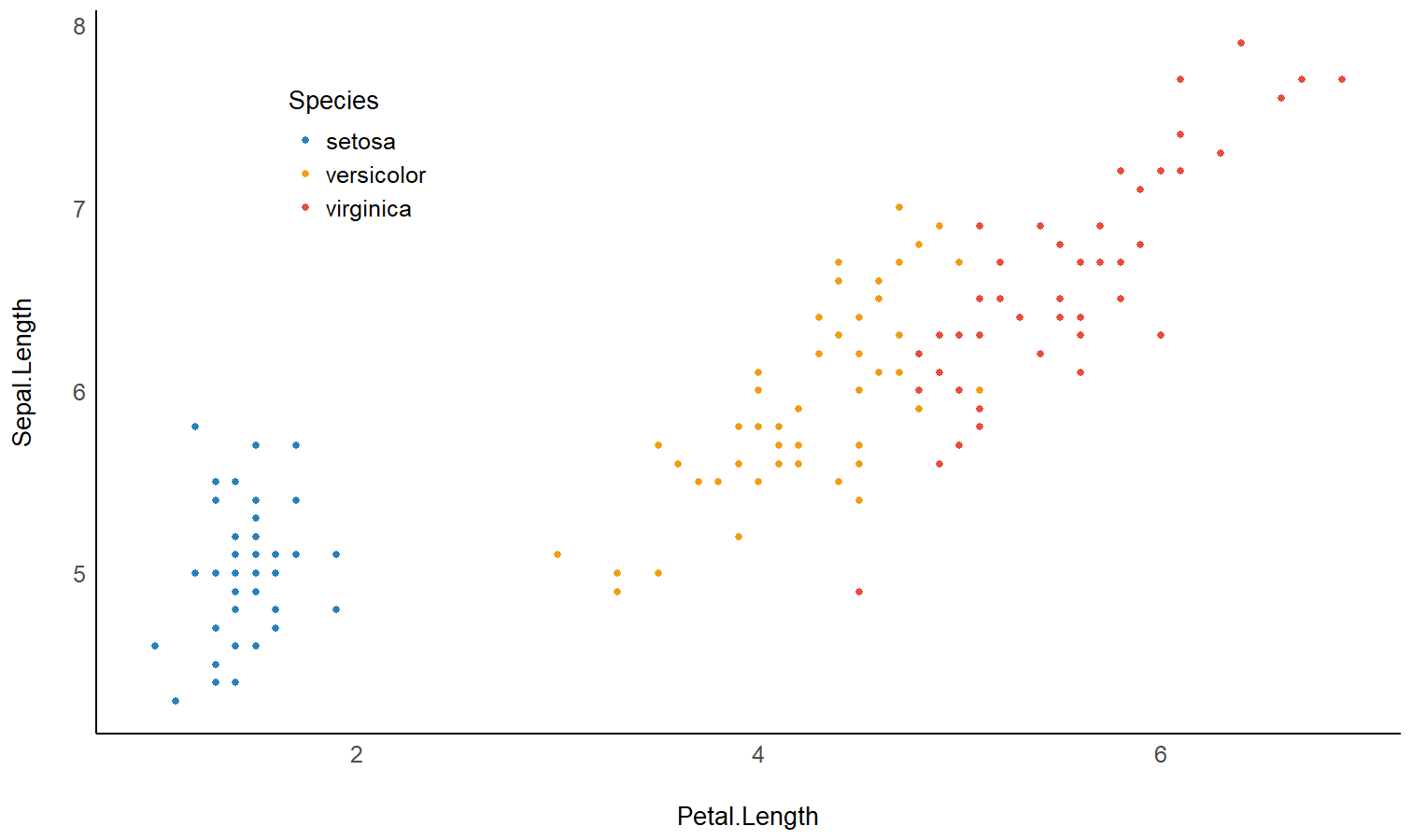Note that these Bayes factors compare the restricted model to the unrestricted model. If we wanted to compare the restricted model to the null model, we could use the transitive property of Bayes factors like so: $BF_{\text{restricted vs. NULL}} = \frac {BF_{\text{restricted vs. un-restricted}}} {BF_{\text{un-restricted vs NULL}}}$ Because these restrictions are on the prior distribution, they are only appropriate for testing pre-planned (a priori) hypotheses, and should not be used for any post hoc comparisons (Morey, 2015). NOTE: See the Specifying Correct Priors for Factors with More Than 2 Levels appendix below. # Bayesian Model Averaging In the previous section, we discussed the direct comparison of two models to determine if an effect is supported by the data. However, in many cases there are too many models to consider, or perhaps it is not straightforward which models we should compare to determine if an effect is supported by the data. For such cases, we can use Bayesian model averaging (BMA) to determine the support provided by the data for a parameter or term across many models. ### Inclusion Bayes factors Inclusion Bayes factors answer the question: Are the observed data more probable under models with a particular predictor, than they are under models without that particular predictor? In other words, on average, are models with predictor $$X$$ more likely to have produced the observed data than models without predictor $$X$$?5 Since each model has a prior probability, it is possible to sum the prior probability of all models that include a predictor of interest (the prior inclusion probability), and of all models that do not include that predictor (the prior exclusion probability). After the data are observed, and each model is assigned a posterior probability, we can similarly consider the sums of the posterior models’ probabilities to obtain the posterior inclusion probability and the posterior exclusion probability. Once again, the change from prior inclusion odds to the posterior inclusion odds is the Inclusion Bayes factor [“$$BF_{Inclusion}$$”; Clyde, Ghosh, & Littman (2011)]. Lets use the brms example from above: bayesfactor_inclusion(comparison) > Inclusion Bayes Factors (Model Averaged) > > P(prior) P(posterior) Inclusion BF > Petal.Length 0.60 1.00 1.91e+25 > Species 0.60 1.00 1.25e+09 > Petal.Length:Species 0.20 0.04 0.171 > > * Compared among: all models > * Priors odds: uniform-equal If we examine the interaction term’s inclusion Bayes factor, we can see that across all 5 models, a model with the term is on average (1/0.171) 5.84 times less supported than a model without the term. Note that Species, a factor represented in the model with several parameters, gets a single Bayes factor - inclusion Bayes factors are given per predictor! We can also compare only matched models - such that averaging is done only across models that (1) do not include any interactions with the predictor of interest; (2) for interaction predictors, averaging is done only across models that contain the main effects from which the interaction predictor is comprised (see explanation for why you might want to do this here). bayesfactor_inclusion(comparison, match_models = TRUE) > Inclusion Bayes Factors (Model Averaged) > > P(prior) P(posterior) Inclusion BF > Petal.Length 0.40 0.96 2.74e+25 > Species 0.40 0.96 1.80e+09 > Petal.Length:Species 0.20 0.04 0.043 > > * Compared among: matched models only > * Priors odds: uniform-equal ### Comparison with JASP bayesfactor_inclusion() is meant to provide Bayes Factors per predictor, similar to JASP’s Effects option. Let’s compare the two: 1. Across all models: library(BayesFactor) data(ToothGrowth) ToothGrowth$dose <- as.factor(ToothGrowth\$dose)

BF_ToothGrowth <- anovaBF(len ~ dose * supp, ToothGrowth, progress = FALSE)

bayesfactor_inclusion(BF_ToothGrowth)
> Inclusion Bayes Factors (Model Averaged)
>
>           P(prior) P(posterior) Inclusion BF
> supp          0.60         1.00       140.99
> dose          0.60         1.00     3.21e+14
> dose:supp     0.20         0.72        10.12
>
> * Compared among: all models
> *    Priors odds: uniform-equal1. Across matched models:
bayesfactor_inclusion(BF_ToothGrowth, match_models = TRUE)
> Inclusion Bayes Factors (Model Averaged)
>
>           P(prior) P(posterior) Inclusion BF
> supp          0.40         0.28        59.19
> dose          0.40         0.28     1.36e+14
> dose:supp     0.20         0.72         2.57
>
> * Compared among: matched models only
> *    Priors odds: uniform-equal1. With Nuisance Effects:

We’ll add dose to the null model in JASP, and do the same in R:

BF_ToothGrowth_against_dose <- BF_ToothGrowth[3:4] / BF_ToothGrowth # OR:
# update(bayesfactor_models(BF_ToothGrowth),
#        subset = c(4, 5),
#        reference = 3)
BF_ToothGrowth_against_dose
> Bayes factor analysis
> --------------
>  supp + dose             : 59  ±4.5%
>  supp + dose + supp:dose : 152 ±1.5%
>
> Against denominator:
>   len ~ dose
> ---
> Bayes factor type: BFlinearModel, JZS
bayesfactor_inclusion(BF_ToothGrowth_against_dose)
> Inclusion Bayes Factors (Model Averaged)
>
>           P(prior) P(posterior) Inclusion BF
> dose          1.00         1.00
> supp          0.67         1.00       105.74
> dose:supp     0.33         0.72         5.06
>
> * Compared among: all models
> *    Priors odds: uniform-equal